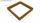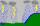# Body volume + rectangle - math problems

#### Number of problems found: 36

• Square prismCalculate the volume of a square prism of high 2 dm wherein the base is: rectangle with sides 17 cm and 1.3 dmCalculate the volume of a quadrilateral pyramid, the base of which has the shape of a rectangle with dimensions a = 6cm, b = 4cm and height v = 11cm
• CylindersThe area of the side of two cylinders is the same rectangle of 33 mm × 18 mm. Which cylinder has a larger volume and by how much?
• Angle of diagonalAngle between the body diagonal of a regular quadrilateral and its base is 60°. The edge of the base has a length of 10cm. Calculate the body volume.The quadrilateral pyramid has a rectangular base of 24 cm x 3.2dm and a body height of 0.4m. Calculate its volume and surface area.
• Building baseExcavation for the building base is 350x600x26000. Calculate its volume in m3.
• Pool in litresPool has a width of 3.5 m length of 6 m and a height 1.60 meters. Calculate pool volume in liters.
• The tankThe tank has 1320 liters of water. The tank has the shape of a prism, its base is an rectangle with sides a = 0,6 m and b = 1,5 m. How high does the water level reach in the tank?
• Surface and volume od cuboidContent area of the square base of cuboid is Sp = 36 cm2 and its height 80 mm. Determine its surface area and volume.
• Uboid volumeCalculate the cuboid volume if the walls are 30cm², 35cm², 42cm²
• Percitipation - meteoDo you have any tip for calc percitipation on a lnd ? Example on 2 ha falls to 5 mm, how many cubic meters is it?
• Cuboid wallsThe base of the block is a rectangle whose sides have lengths in the ratio 13: 7. Find the volume of the block in liters, if the longer side of the base measures 65 cm and the height of the block is 1.2 m
• Prism 4 sidesFind the surface area and volume four-sided prism high 10cm if its base is a rectangle measuring 8 cm and 1.2dm
• Cuboid - volume and areasThe cuboid has a volume of 250 cm3, a surface of 250 cm2 and one side 5 cm long. How do I calculate the remaining sides?
• Cylinder - basicsCylinder with base radius r = 45 dm and height h=22 dm. Calculate:
• Tetrahedral pyramid 8Let’s all side edges of the tetrahedral pyramid ABCDV be equally long and its base let’s be a rectangle. Determine its volume if you know the deviations A=40° B=70° of the planes of adjacent sidewalls and the plane of the base and the height h=16 of the p
• RainfallHow many liters of water did fell in a 32m long and 8m wide garden, if 8mm of rain fell?
• Triangular prismCalculate the volume and surface area of a triangular prism if it is given: a = 6.8 dm. ..Va = 4 dm. (base edge length and base triangle height length) ... ... .v = 23 dm (body height)
• Prism - boxThe base of prism is a rectangle with a side of 7.5 cm and 12.5 cm diagonal. The volume of the prism is V = 0.9 dm3. Calculate the surface of the prism.
• Annual rainfallThe average annual rainfall is 686 mm. How many liters will fall on the 1-hectare field?

Do you have an interesting mathematical word problem that you can't solve it? Submit a math problem, and we can try to solve it.

We will send a solution to your e-mail address. Solved examples are also published here. Please enter the e-mail correctly and check whether you don't have a full mailbox.

Please do not submit problems from current active competitions such as Mathematical Olympiad, correspondence seminars etc...

Body volume - math word problems. Rectangle Problems.